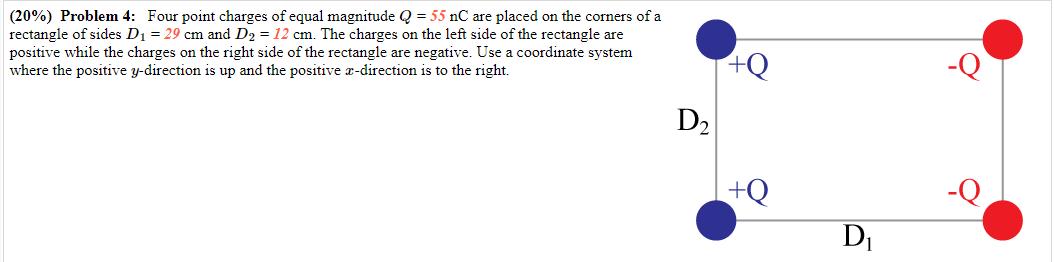Home / Expert Answers / Physics / part-a-calculate-the-vertical-component-of-the-net-force-in-newtons-on-the-charge-which-lies-a-pa846

# (Solved): Part a - Calculate the vertical component of the net force, in newtons, on the charge which lies a ...Part a - Calculate the vertical component of the net force, in newtons, on the charge which lies at the lower left corner of the rectangle.

Part b - Calculate the magnitude of the net force, in newtons, on the charge located at the lower left corner of the rectangle.

Part c - Calculate the angle of the net force vector relative to the +x axis, with the positive direction being counterclockwise from the positive horizontal axis. Enter an angle between -180° and 180°